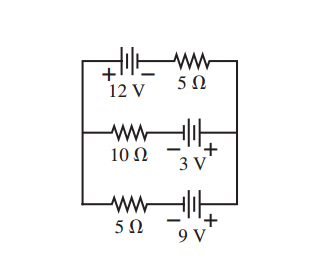# [SOLVED] What is the current through the 10 Ω resistor in the figure?

What is the current through the 10 Ω resistor in the figure?I tried (12/5)-(9/5)-(3/10) but it gives the wrong answer

the answer is suppose to be 0.36 A

selected by

## The Best answer for What is the current through the 10 Ω resistor in the figure?

Use Thevenin's theorem to represent the 9v and 12v sources and their series resistors as a single voltage source in series with a single resistor.

Because those two sources are in series with the same value of resistor, the equivalent voltage is (1/2)*(9 + (-12)) = -1.5v.

The equivalent resistance is two 5 ohms in parallel, ie 2.5 ohms.

When that source is connected to the 3v source and its series resistor, the current is (3 + 1.5)v/(10 + 2.5) ohms = 0.36

amps

Lorem ipsum dolor sit amet, consectetur adipiscing elit, sed do eiusmod tempor incididunt ut labore et dolore magna aliqua. Ut enim ad minim veniam, quis nostrud exercitation ullamco laboris nisi ut aliquip ex ea commodo consequat. Duis aute irure dolor in reprehenderit in voluptate velit esse cillum dolore eu fugiat.

because it is voltage gain

or

Why do you add the 1.5V's to the 3V instead of subtracting them since the one of the equivalent voltage is -1.5.

Lorem ipsum dolor sit amet, consectetur adipiscing elit, sed do eiusmod tempor incididunt ut labore et dolore magna aliqua. Ut enim ad minim veniam, quis nostrud exercitation ullamco laboris nisi ut aliquip ex ea commodo consequat. Duis aute irure dolor in reprehenderit in voluptate velit esse cillum dolore eu fugiat.

+1 vote
+1 vote